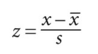# Z-value

(redirected from Z value)

## Z-value

(in statistics) indicates how many STANDARD DEVIATIONS away from the MEAN a value resides. For a given set of data the mean (x) is subtracted from each member (x) of the set; then each member is divided by the standard deviation (s) of the set: z = (x - x)/s. A positive score indicates the value is above the mean, a negative score indicates below. Z-values are useful in comparing sets of data with different means and standard deviations.Collins Dictionary of Biology, 3rd ed. © W. G. Hale, V. A. Saunders, J. P. Margham 2005
Site: Follow: Share:
Open / Close